# Maximum Subarray Sum

Difficulty: Medium, Asked-in: Facebook, Microsoft, Amazon, Morgan Stanley, Walmart, Flipkart, Hike, Oracle, Samsung, Snapdeal, Zoho

Key takeaway

• This is one of the best interview problems to learn step-by-step optimization using several problem-solving approaches.
• Kadane algorithm idea is intuitive, using a single loop and few variables to solve the problem. We can use a similar idea to solve other coding problems.

### Let’s understand the problem

Given an array X[] with n elements, we need to write a program to find the maximum sum of a subarray among all subarrays. A subarray of array X[] is a contiguous segment from X[i] through X[j] where 0<= i <= j <= n.

• If the array contains all non-negative numbers, the maximum subarray sum would be the sum of the entire array.
• Several different sub-arrays may have the same max sum but we need to just return the value of the max subarray sum.

Example 1

Input: X[] = [-4, 5, 7, -6, 10, -15, 3], Output: 16

Explanation: The subarray [5, 7, -6, 10] has the maximum sum.

Example 2

Input: X[] = [-3, 2, -1, 4,-2], Output: 5

Explanation: The subarray [2, -1, 4] has the maximum sum.

Example 3

Input: X[] = [5, 7, 6, 10, 3], Output: 31

Explanation: All array elements are non-negative. So the maximum subarray sum would be the sum of the entire array.

### Discussed solution approaches

• A brute force approach  using three nested loops
• Using two nested loops
• Using divide and conquer approach similar to the merge sort
• Using dynamic programming
• Kadane algorithm: Using single loop and variables

### A brute force approach  using three nested loops

The most basic solution is to explore all the possible subarray for all i and j where i≤j. We calculate the sum of each subarray and return the maximum among them.

Solution Pseudocode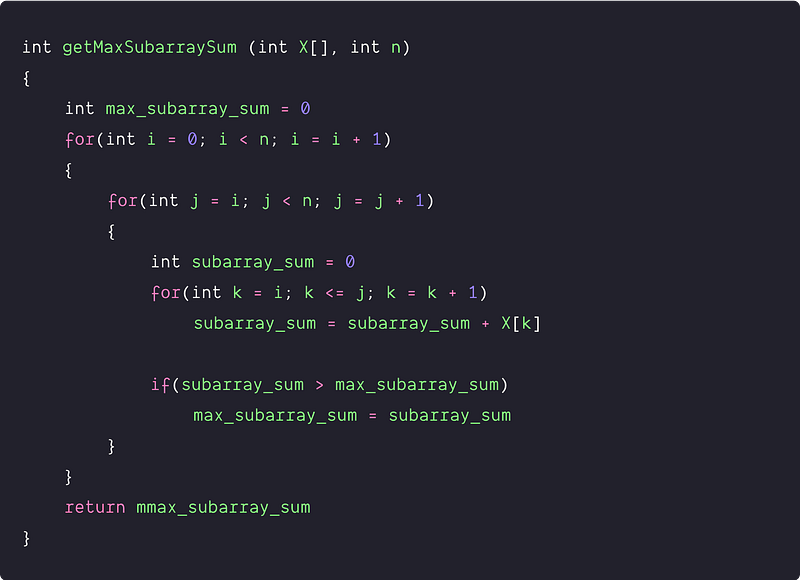Time and space complexity analysis

We are using three nested loops : exploring all pairs in the outer two loops and calculating the running sum in the inner loop. Time complexity = O (n³). Space complexity = O(1)

### Using two nested loops

Solution Idea

Now the critical question would be : can we optimize the above approach further? is it necessary to use the inner loop? Let’s think!

If we observe closely, we can calculate the sum of the sub-array from i to j+1 easily if we know the sum of the sub-array from i to j. So there is no need for an innermost loop to calculate the sum every time for each pair of (i, j). We can calculate the subarray sum using this formula Sum(i, j+1) = Sum(i, j) + X[j+1]. Using this formula, for every value of i, we can calculate the running sum for all values of j.

Solution Pseudocode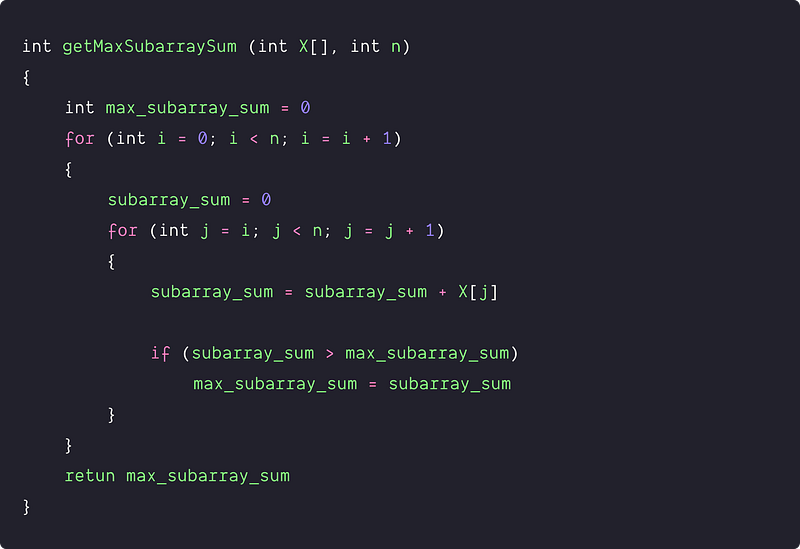Time and space complexity analysis

There are only two nested loops in the above code. Time complexity = O(n²), Space complexity = O(1)

### Using divide and conquer idea similar to merge sort

Again we should ask the same question: Can we improve the time complexity further? can we solve this problem using smaller sub-problems or the Divide & Conquer approach? Let's think!

Solution Idea

Suppose we want the maximum sub-array sum of the array X[l…r]. Let's divide the array into two equal subarrays — X[l…mid] and X [mid+1…r]. Then the maximum continuous sub-array X[i…j] must lie in one of the following places:

• Entirely in the left sub-array X[l…mid], where l ≤ i ≤ j ≤ mid.
• Entirely in the right sub-array X[mid+1…r], where mid+1 ≤ i ≤ j ≤ r
• Subarray crossing the mid-point, so that low ≤ i ≤ mid ≤ j ≤ r. This includes some rightmost elements of the left subarray and some leftmost elements of the right subarray.

We can find the maximum sub-arrays of X[l…mid] and X[mid+1…r] recursively because these two sub-problems are smaller instances of the problem of finding a maximum sub-array sum. So now we need to calculate the maximum sub-array that crosses the mid-point and takes the maximum of all three possibilities.

Solution Pseudocode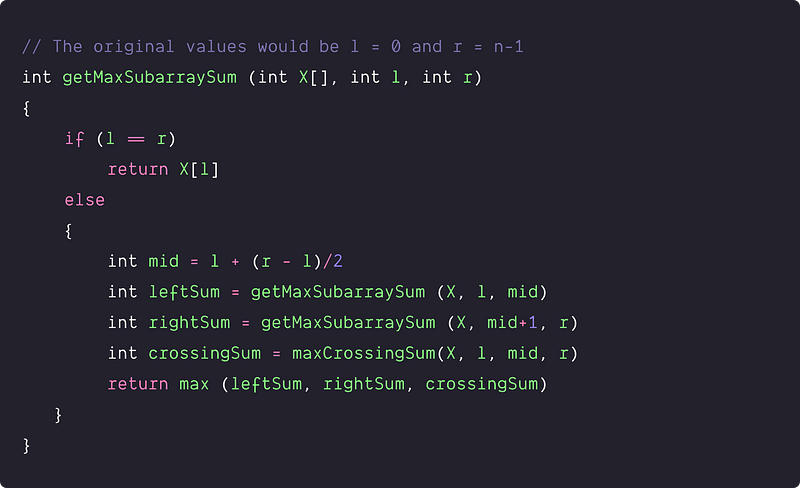Combine part  implementation:  maxCrossingSum(X, l, mid, r)

Sub-array crossing the mid-point comprises two sub-arrays, X[i…mid] and X[mid+1…j]. So we need to find the maximum sub-array form X[i…mid] and X[mid+1…j] and then combine them.

This idea works as follows:

• First, we find the maximum subarray sum of the left half X [l…mid]. Since this subarray must contain X[mid], so we run a loop starting from the index i = mid to l and considers every subarray of form X[i…mid].
• We initialize a variables max_leftSum, which holds the maximum sum found so far in the left half, and variable sum containing the sum of the entries in X[i…mid].
• Whenever we find a subarray X [i…mid] with a sum of values greater than maxleftSum, we update maxleftSum to this subarray’s sum.
• Similarly, for the right half X[mid+1, r], we run a loop starting from the index j = mid+1 to r and considers every subarray of form X[mid+1…r]. We initialize and store the maximum sum found so far in the right half in the variable max_rightSum.
• Finally, we add maxleftSum + maxrightSum and return this value.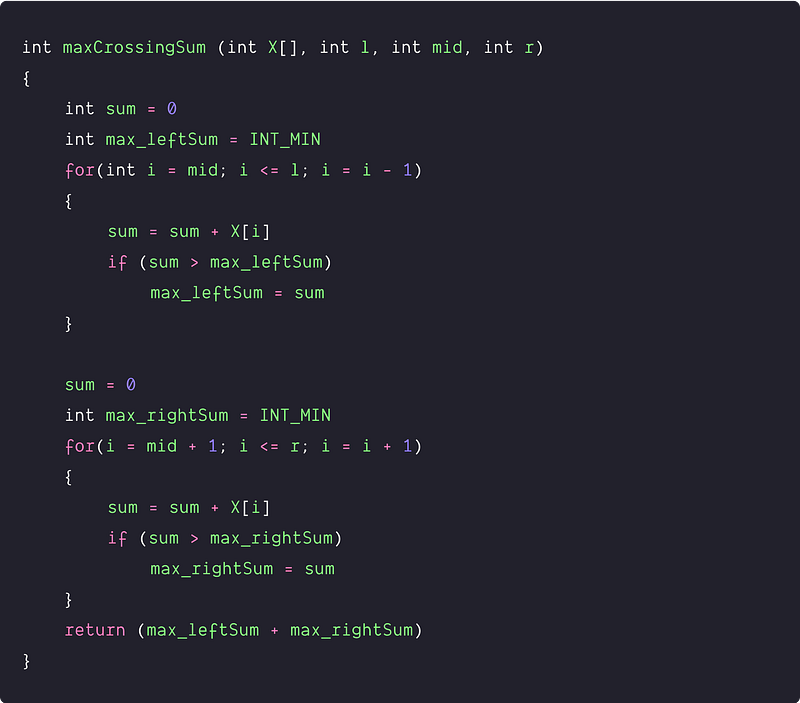Time and space complexity analysis of divide and conquer approach

For simplifying the analysis, we assume that the original problem size is a power of 2, and T(n) is the time complexity of the max subarray of input size n. To calculate the overall time complexity, we need to add the time complexities of the divide, conquer, and combine parts.

• For the base case (n=1), the algorithm takes constant time. T(1) = O(1). The recursive case occurs when n>1.
• Time Complexity of divide part = O(1) because we need to calculate the mid to divide the array into two equal halves.
• The time complexity of the conquer Part: The input size of each subproblem is n/2 elements, so we spend T(n/2) time solving each. We need to solve two subproblems of size n/2, so the overall time complexity of solving smaller subproblems is 2T(n/2).
• The time complexity of the combine part: Two separate single loops are running in the opposite direction, wherein in the worst case, each loop runs n/2 times. So time complexity of this stage is O(n).

Final recurrence relation: T(n) = O(1), if n=1, T(n) = 2 T(n/2) + O(n), if n>1

This recurrence is the same as a recurrence for merge sort. As we shall see from the master method, this recurrence has the solution T(n) = O(n logn). You might also revisit the recursion tree method of merge sort to understand why the solution should be O(n logn).

Space complexity = O(logn), for the stack space by recursive calls (Think!)

### Using dynamic programming

Solution Idea

Let’s take an array maxSumEnd[] of size n, where each maxSumEnd[i] denotes maximum subarray sum ending at index i. Now if we know the maxSumEnd[i-1], then we can easily calculate the maxSumEnd[i]. Here are insights:

• maxSumEnd[i] always include the value X[i].
• If (maxSumEnd[i -1] > 0), then we should include maxSumEnd[i -1] to calculate maxSumEnd[i] because X[i] < maxSumEnd[i-1] + X[i]. So in this situation, maxSumEnd[i] = maxSumEnd[i-1] + X[i]
• If (maxSumEnd[i -1] < 0), then we should ignore maxSumEnd[i -1] to calculate maxSumEnd[i] because X[i] > maxSumEnd[i-1] + X[i]. So in this situation, maxSumEnd[i] = X[i]
• In more simple way, we can write, maxSumEnd[i] = max (maxSumEnd[i-1] + X[i], X[i])

Finally, we return the maximum value stored in the maxSumEnd[] array to find the solution. This problem falls under the dynamic programming strategy because we store the solution of sub-problems and solve the larger problem using the solution of a smaller sub-problem.

Solution Steps

1. Create an extra array maxSumEnd[] of size n
2. Initialize the array with basic solution i.e. maxSumEnd = A
3. Traverse the array from i = 1 to n-1, and any ith iteration pick current element X[i] and calculate the maxSumEnd[i].
4. If (maxSumEnd[i-1] < 0) then maxSumEnd[i] = X[i]
5. Else, maxSumEnd[i] = X[i] + maxSumEnd[i-1]
6. Return the maximum of sum stored in maxSumEnd[n] array.

Solution Pseudocode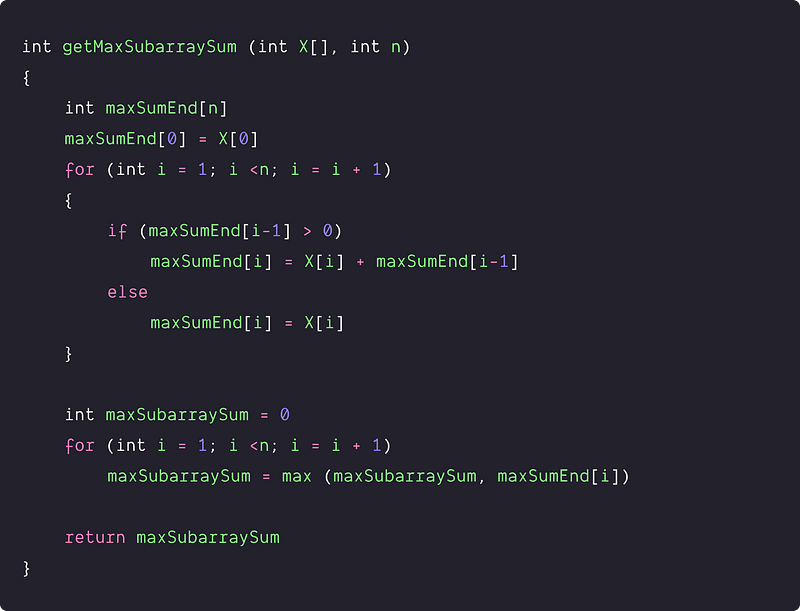Time and space complexity analysis

We are traversing the array twice using a single loop. So time Complexity = O(n). Space Complexity = O(n), for the extra array maxSumEnd[n]

### An efficient approach using the Kadane algorithm

Solution Idea

Now the critical question is: can we solve the problem in O(1) space? Furthermore, can we solve the problem in a single traversal? Here is the optimized solution using the famous kadane algorithm:

• According to the above approach, we can easily calculate the max subarray sum ending at the ith index using a single loop. Let’s store this value in a variable maxSum_endingHere.
• The maximum subarray sum will be the maximum of all the subarray sums ending at index i. So we can track the maximum subarray sum in a variable maxSumsofar.
• During each iteration, if (maxSumendingHere > maxSumsofar) then we update maxSumsofar = maxSumendingHere.
• By the end of the loop, the maximum subarray sum value gets stored in the variable maxSumsofar. We return this value as an output.

In the above idea, we are using two extra variables and one traversal. No extra space, No double traversal! It's an excellent optimization.

Solution steps

1. We initialize the variable maxSumsofar and maxSum_endingHere with X.
2. We run a loop from i = 1 to n -1 where at each ith iteration.
3. Calculate max subarray sum ending at the ith index in i.e maxSumendingHere = max (maxSumendingHere + X[i], X[i])
4. Update maxSumsofar i.e. if(maxSumsofar < maxSumendingHere) then maxSumsofar = maxSumendingHere
5. ﻿By end of the loop we return maxSumsofar

Solution Pseudocode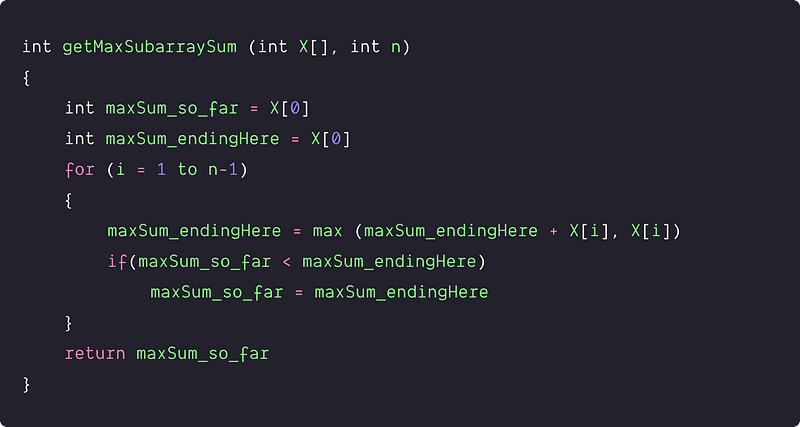Time and space complexity analysis

Each element has been visited only once using a single loop. We are also doing constant operation at each step of iteration of the loop. So time complexity = O(n). Space complexity = O(1), we are using a constant number of variables only.

### Critical ideas to think!

• How can we optimize the above algorithms to find the start and end indices of the sub-array with maximum sum?
• What is the time complexity of the best solution if the sorted array is provided as an input for this problem?
• Can we use the same approach to find the max product subarray?
• Do the above algorithms handle the case of negative numbers?

### Comparisons of time and space complexities

• Three nested loops: Time = O(n^3), Space = O(1)
• Two nested loops: Time = O(n^2), Space = O(1)
• Divide and Conquer: Time = O(nlogn), Space = O(logn)
• Dynamic Programming: Time = O(n), Space = O(n)
• Kadane Algorithm: Time = O(n), Space = O(1)

### Similar coding questions to practice

• Find the maximum product subarray
• Find the sum of the maximum elements of every possible sub-array
• Calculate maximum sum submatrix of size k x k in m x n matrix
• The longest subarray in a binary array with an equal number of 0s and 1s
• Largest sum contiguous subarray having only non-negative elements

Enjoy learning, Enjoy coding, Enjoy algorithms!

Get well-designed application and interview centirc content on ds-algorithms, machine learning, system design and oops. Content will be delivered weekly.